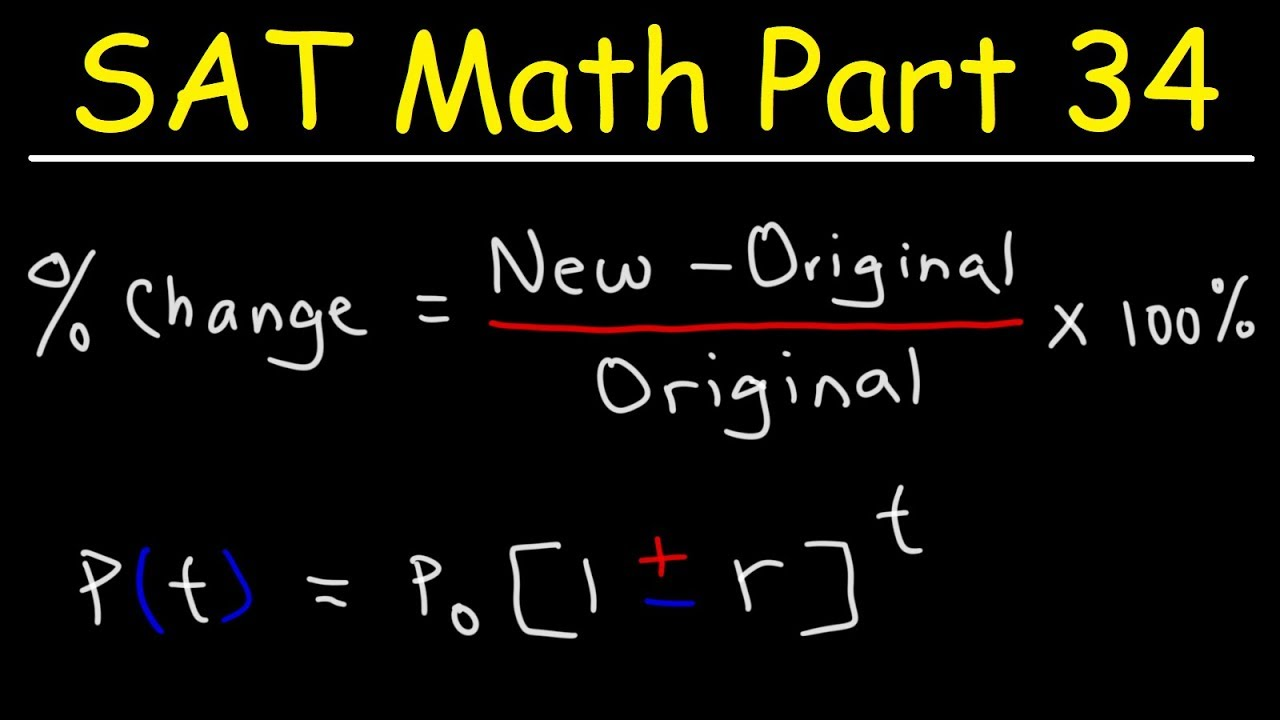By using our services, you agree to our Privacy Policy. Click to accept.

# Percent Increase & Decrease, Population Growth Problems - SAT Math Part 34

This SAT Math video tutorial provides a basic introduction into calculating the percent increase and decrease of an event using the percent change formula. It also explains how to solve word problems involving population growth and how to write functions that will help you to calculate the future population value.

Here are some other useful links:

SAT Math Part 35:

SAT Chemistry Part 1:

Chemistry Final Exam Review:

Algebra Final Exam Review:

Geometry Final Exam Review:

Physics Final Exam Review:

Trigonometry Final Exam Review: